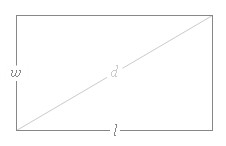## Rectangle Calculator

 Width: Length: Area: Perimeter: Diagonal: Note: Fill in any two items and get the results of other three.

In plane geometry, A rectangle is a four-sided flat shape. rectangle's Each internal angle is 90°. Opposite sides of rectangle are parallel and of equal length.

### Rectangle formula:

Area = w × L

Perimeter = 2(w+L)

Diagonal "d" = √(w2 + L2)For example, When width =2, length = 3, the area = 6, perimeter = 10, diagonal = 3.6056

For example, When width =2, perimeter = 20, the length = 8, area = 16, diagonal = 8.2462

Thinkcalculator.com provides you helpful and handy calculator resources.